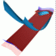Abstract and Contents
Up: 5. Examples
Next: 5.4 The Lorenz system
Previous: 5.2 Quasiperiodically forced Hénon map

# 5.3 A saddle surface

The computation of the two-dimensional stable or unstable manifold of a hyperbolic fixed point typically requires adding leaves during the computation. This is illustrated with the example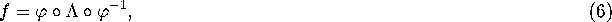where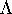is a diagonal matrix and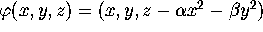. The origin is a fixed point of f. The eigenvalues of its linear part are the eigenvalues ofand the corresponding eigenvectors are the coordinate axes. We take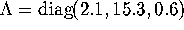,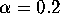and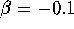. Due to the special form of (6), the two-dimensional unstable manifold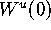of the origin is the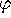-image of the-plane. Since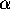and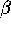have opposite signs, this unstable manifold is a saddle surface.

We computedwith our algorithm; see Figure 13. The global foliation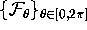is chosen as described in Section 3.3. The linear approximation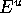of the unstable manifold is the-plane. Each leaf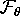ofis the plane perpendicular tothat intersectsin a ray with argument. We start the computations with a ring inthat is bounded by two circles centered at

the origin with radii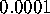and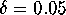. The mesh on this first approximation is formed by the intersection of this ring with 40 leaves of. If the distance between points on two neighboring leaves exceeds, we add an additional leaf. We take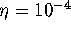for the bisection error. The outer circle in Figure 13 has an (arclength) radius of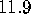and consists of 496 mesh points. During the globalization process, the width of the computed rings does not exceed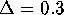. The colored rings in Figure 13 have width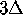.

The regularity of the mesh thus obtained is shown in Figure 14 (right), whereas Figure 14 (left) shows the mesh for the same manifold computed with a fixed set of 40 leaves. Because there is an explicit formula for the global unstable manifold of (6), we can compare it with the numerical approximation. Every point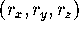on the approximation corresponds to a point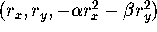on the actual manifold, so that the error is the difference between the respective z-coordinates. We compute the error at the mesh points and at the midpoints between two neighboring points on different leaves. This error is an indication of the global error.

circle radius error fixed mesh error adapted mesh
 0 0 0 0 1 4 7 10 11 . . . . . . . . . 05 1 2 5 1 1 1 1 9
 0 0 0 0 0 0 0 0 0 . . . . . . . . . 0005 0005 0004 0014 0021 0204 0696 1612 2420
 0 0 0 0 0 0 0 0 0 . . . . . . . . . 0005 0005 0004 0014 0021 0047 0057 0051 0053

Table 1: The error between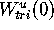and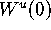for the saddle surface in Figure 13. The error at circles with the given (arclength) radii is the maximum error at the meshpoints and at the midpoints between the mesh points.

The error on circles with increasing (arclength) radius is shown in Table 1. For the first four steps the minimum distance of the circle to its image is smaller than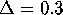. As this distance increases, the error increases. For distances larger than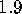the algorithm with mesh adaptation (right column) starts adding leaves. Because the saddle surface is, the error in Table 1 stays small compared to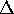.

Up: 5. Examples
Next: 5.4 The Lorenz system
Previous: 5.2 Quasiperiodically forced Hénon mapAbstract and Contents

Written by: Bernd Krauskopf & Hinke Osinga
Created: May 27 1997 --- Last modified: Fri May 30 21:40:51 1997